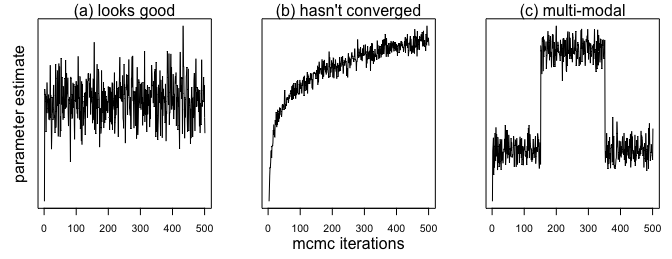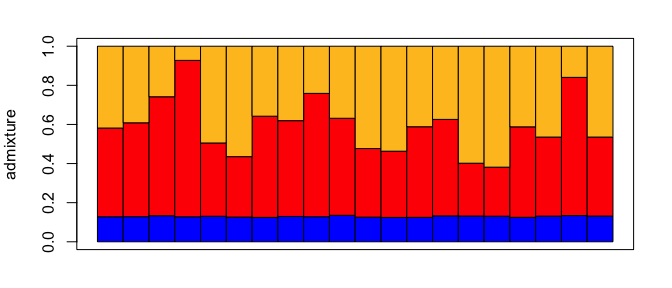# How to run a conStruct analysis

## Run conStruct

This document describes how to run a conStruct analysis.

Throughout the document, I’ll be referring to the example dataset included with the package:

library(conStruct)
data(conStruct.data)

The format for the data you need to run a conStruct analysis is covered in a separate vignette in this package. You can view that vignette using the command: vignette(package="conStruct",topic="format-data"). If you’ve already run conStruct and you want more information on how to visualize the results, please see the companion vignette for visualizing results. If you’ve run several conStruct analyses and want to compare them, please see the companion vignette for model comparison.

## Running a conStruct analysis

The function you use to run a conStruct analysis is called, fittingly, conStruct. This vignette walks through the use of this function in detail, and should be used in concert with the documentation for the function, which can be viewed using the command: help(conStruct).

### Spatial Model

The default model in the conStruct package is the spatial model, which allows relatedness within a layer to decay as a function of the distance between samples drawing ancestry from that layer.

Below, I show an example of how to run a conStruct analysis using the spatial model.

# load the example dataset
data(conStruct.data)

# run a conStruct analysis

#   you have to specify:
#       the number of layers (K)
#       the allele frequency data (freqs)
#       the geographic distance matrix (geoDist)
#       the sampling coordinates (coords)

my.run <- conStruct(spatial = TRUE,
K = 3,
freqs = conStruct.data$allele.frequencies, geoDist = conStruct.data$geoDist,
coords = conStruct.data$coords, prefix = "spK3") The function call above runs conStruct’s spatial model using 3 discrete layers. All output files will be have “spK3” prepended to their names. To vary the number of layers in the spatial model, you need only change the value of K. The example dataset conStruct.data is organized into an R list for convenience, but users can provide their data to the function any way they see fit, so long as each argument is properly formatted (e.g., freqs is a matrix, prefix is a character vector, etc.). ### Nonspatial Model You can also run a nonspatial model using the conStruct function, in which relatedness within each of the K clusters does not decay with distance. This model is analogous to the model implemented in STRUCTURE. Below, I show an example of how to run a conStruct analysis using the nonspatial model. # load the example dataset data(conStruct.data) # run a conStruct analysis # you have to specify: # the number of layers (K) # the allele frequency data (freqs) # the sampling coordinates (coords) # # if you're running the nonspatial model, # you do not have to specify # the geographic distance matrix (geoDist) my.run <- conStruct(spatial = FALSE, K = 2, freqs = conStruct.data$allele.frequencies,
geoDist = NULL,
coords = conStruct.data$coords, prefix = "nspK2") The function call above runs conStruct’s nonspatial model using 2 discrete layers. All output files will be have “nspK2” prepended to their names. As with the spatial model, if you want to vary the number of layers, you change the value of K. ### Other function options The conStruct function has other arguments that have default values, for which you don’t have to specify any values. However, you may wish to alter these defaults, so we describe them below: The full function call for the spatial model with 3 layers is: my.run <- conStruct(spatial = TRUE, K = 3, freqs = conStruct.data$allele.frequencies,
geoDist = conStruct.data$geoDist, coords = conStruct.data$coords,
prefix = "spK3",
n.chains = 1,
n.iter = 1000,
make.figs = TRUE,
save.files = TRUE)

The other options are n.chains, n.iter, make.figs, save.files; I describe each of them below:

• n.chains - gives the number of independent MCMCs to be run for this model. The default is 1, but you may wish to run multiple independent chains to make sure you get consistent results across them.

• n.iter - gives the number of iterations per MCMC. The default is 1000. If you have more genotyped samples, you generally need more iterations to describe the posterior probability surface well. There are no hard and fast rules on how many iterations you should run. I strongly recommend examining model output to assess convergence; if you don’t see good convergence, you can run the analysis using a larger number of iterations.

• make.figs - determines whether or not to automatically make figures describing the results. The default is TRUE. However, if you’re running lots of independent analyses, or if you’re running on a cluster with limited disk space, you may wish to set this option to FALSE and make the figures later on your own.

• save.files - determines whether or not to automatically save all output files. The default is TRUE. However, again, there may be circumstances in which you don’t want to automatically save these files, and instead want to capture the results of the analysis, which are the returned value of the conStruct function call.

## Model diagnosis

As with any statistical model, it is important to assess the performance of the inference method. Below, I briefly walk through some of the important things to look out for when you run a conStruct analysis.

### MCMC diagnosis

Although the Hamiltonian Monte Carlo algorithm implemented in STAN is quite robust, it’s always a good idea to look at the results of the analysis to diagnose MCMC performance. If the chain is mixing well, the trace plots for the different parameters and the posterior probability will resemble a “fuzzy caterpillar,” as in panel (a) below. If the trace plots have not plateaued (as in panel (b)), it is an indication that the chain has not converged on the stationary distribution, and that it should be run longer. If the chain appears to be bouncing between two or more modes, as in panel (c) below, that may be an indication of a multi-modal likelihood surface, with multiple points in parameter space that have equal or similar posterior probability given the data.### Independent runs

Above, I highlight the importance of evaluating performance of individual MCMC runs, but it’s also a good idea to run multiple, independent analyses and compare results across them. Ideally, multiple independent runs converge on the same stationary distribution, with similar parameter estimates and posterior probabilities.
If different runs give very different results, you can check whether there’s a mixing problem or a truly multi-modal posterior probability surface by comparing the values of the posterior probability across runs. If two runs have very different parameter estimates but their posterior probability distributions are indistinguishable, that’s an indication of multi-modality. If multiple runs show different parameter estimates, but the posterior probabilities for a subset of the runs that show consistent results are higher than those of a different subset that gives conflicting results, that indicates that some of the runs are not mixing well.

### Missing data

Missing data can affect the sample allelic covariance, and therefore the results of a conStruct analysis. This is especially the case when the distribution of missing data is biased - that is, when individuals of particular ancestry are more likely to be missing data at a locus. This pattern is expected when, for example, allelic dropout occurs in a RADseq dataset.

In some empirical datasets with missing data that I used to test conStruct, I observed a phenomenon of “homogeneous minimum layer membership,” (HMLM) in which all samples had troublingly similar admixture proportions in a particular cluster (see membership in the blue layer in the figure below).Users are advised to check the results of their analyses carefully for this HMLM behavior. If you encounter this issue, try reducing the amount of missing data in your dataset, either by dropping poorly genotyped samples or poorly genotyped loci (rows and columns of the allele frequency data matrix, respectively).